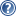###LON-CAPA Help

This section describes the layout of each level of spreadsheet. See the section on the Spreadsheet Hierarchy () for a description of the types of spreadsheets and their data-flow relationships.

General Characteristics

Each spreadsheet has 52 columns and a variable number of rows. The columns of the spreadsheets are labeled 'A-z'. The rows of the spreadsheets contain data appropriate to the spreadsheet as described below. The section Spreadsheet Row Numbers () describes how row numbers are assigned.

Assessment Spreadsheet Layout

There are two special rows on the top of the assessment spreadsheet. The first is the template row (). The second row is the export row ().

The main body of the assessment spreadsheet contains the parameters associated with the assessment. The parameter values appear in the "A" column of the spreadsheet and cannot be edited. The remainder of the spreadsheet columns, labeled "B-z", can be edited and used for computations. See Referencing Spreadsheet Parameters () for information on how to reference a parameter value in the spreadsheet.

Student Spreadsheet Layout

The student spreadsheet has both a template row () and an export row ().

The main body of the student spreadsheet contains the export rows of the assessments in the course. Each assessment appears beneath the title of the folder or sequence which it belongs to. The first 26 columns of the main body of the spreadsheet, "A-Z", are the export data from the assessments and cannot be edited. The remaining columns, "a-z", can be edited.

Course Spreadsheet Layout

The course spreadsheet contains the data exported from each student level spreadsheet.

The two topmost rows are the template row () and a summary row. The remainder of the spreadsheet it taken up with student data. Each student spreadsheet exports one row to the course spreadsheet. The first 26 columns, "A-Z", contain the student data and cannot be edited. The remaining 26 columns "a-z", can be edited.